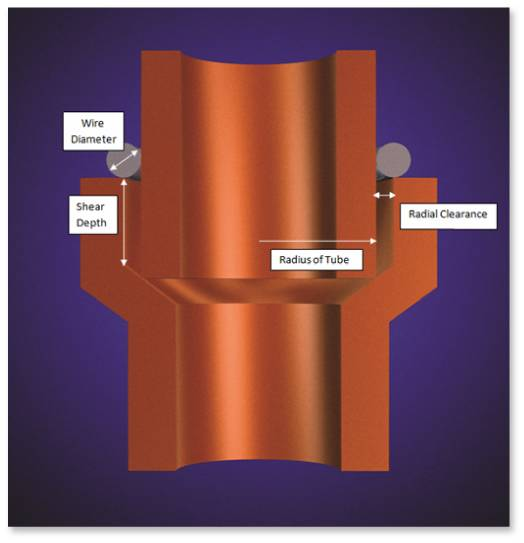Importance of Ring Volume
One factor that may impact the strength of a brazed joint is the depth of alloy penetration into the joint.  If an operator does not apply enough alloy to the assembly, the final part will not have the ideal strength and/or leak tightness characteristics for a brazed joint.

Operators may have the ability to calculate the amount of alloy required to fill their specific joints, but in hand-feeding applications, it is difficult to consistently apply the same amount of alloy every time.  A group of operators may represent varied skill levels, which can increase the chance of inconsistencies in an operation.

To produce a proper brazing joint, the volume of alloy used must match or exceed the volume of the joint to be brazed. Excess alloy adds cost and may lead to an additional post-machining operation if the alloy flows too far outside of the joint.  Lucas-Milhaupt has developed braze alloy rings of pre-measured volumes for each specific application.  These are available in various forms.

Calculating Ring Volume
To determine the proper ring volume for a tube-and-fitting application, the volume of the joint must first be calculated with its widest possible clearance.Each component in a braze assembly has a separate machined tolerance.  You must ensure that the braze alloy can fill the shear depth, regardless of where the components fit within their tolerances.  Thus, it is important to measure for the worst-case scenario.

The inner-dimension (I.D.) value of the fitting in this calculation should be the largest-possible value within tolerance, and the outer-dimension value (O.D.) of the tube in this application should be the smallest-possible value within tolerance.  This will produce the largest possible radial-clearance value.  The volume of the joint, as shown in Figure 1, is the product of the shear depth, radial clearance, and circumference of the tube. A very simple approach to calculating the volume is shown below.

Joint Volume = SD * 2πRtube * Cm

Where:
SD = Shear depth
2πRtube  = Circumference of the tube
Cm = Maximum Radial Clearance = (IDmaxFitting - ODminTube)/2

The volume of the ring for this application will equal the volume of the joint plus a fifteen-percent safety factor to account for fillet and variation in surface roughness, as shown below.

(1.15)Joint Volume = Ring Volume

The diameter of the braze alloy wire needed to make a ring can be calculated using the equation below.  The wire diameter is the only unknown variable in the equation, making it the value for which we are solving.  In calculating the wire diameter, the O.D. of the tube is used as the I.D. of the ring.

Ring Volume = (πDwire^2)/4 * [2π(Rtube)]

Where:
Dwire = wire diameter
(πDwire^2)/4 = Cross-sectional area of wire
2π(Rtube) = OD circumference of tube including cross-sectional radius of wire

To illustrate the calculations, an example of a tube to fitting joint is shown using the following data:

SD = .500in
OD tube = .254in ± .002in
ID fitting = .260in ± .002in

The dimensions that would make the joint clearance largest are used in the equation. Looking at the sample data, the IDmax of the fitting is .262in and the ODmin of the tube is .252in. These dimensions yield the larges joint clearance. The maximum radial clearance used would be half of the IDmax of the fitting minus the ODmin of the tube. In calculating the circumference of the tube, the radius of the tube used is .127in without taking the tolerance into account. The calculation for joint volume is shown below.

SD = .500in
Cm = (.262in -.252in)/2 = .005in

Joint Volume = SD * 2πRtube * Cm
Joint Volume = .500in * 2π(.127in) * .005in
Joint Volume = .002in3

(1.15)Joint Volume = Ring Volume
Ring Volume = (πDwire^2)/4 * [2π(Rtube)]
(1.15) * .002 in = (πDwire^2)/4 * [2π(.127in)]
(4/π) * [(1.15) * .002in] / [2π(.127in)] = Dwire^2
Dwire = .061in

The ring will have a wire diameter of .061in and a ring ID of .254in. The ID of the ring will equal the OD of the tube it goes around.

Conclusion
The depth of alloy penetration into a joint is one factor impacting strength and/or leak tightness of a brazed joint.  To produce a proper brazing joint, the volume of alloy used must match or exceed the volume of the joint to be brazed.  Calculating the volume of the joint clearance will determine the proper ring volume.  Lucas-Milhaupt braze alloy rings, with pre-measured volumes for each specific application, will help ensure consistency and reduce material waste.

For more information on better brazing products or services, contact Lucas-Milhaupt. For further information on preform design and various types of braze preforms, please read previous blog posts: Designing for Preforms-Introduction or Designing for Preforms-Selecting the Brazing Preform.

Topics: Brazing Tips